"Percentages Calculator" App Analytics: ASO Keyword Monitoring | ASOTools

# "Percentages Calculator" App Analytics: ASO Keyword Monitoring | ASOTools

Are you doing Percentages Calculator app analytics for better app store ASO strategies? Whether Percentages Calculator in app store keep on top? What keyword tactics Percentages Calculator take to rank well in app store? Are app store keywords like "math pourcentages", "%", "percentages calculator" matter in Percentages Calculator ranking?

Free ASO tools for Google Play & App Store, ASOTools will reveal the app store keywords secrets of Percentages Calculator.

## App Analytics: Percentages Calculator

Percentages Calculator, published by Thomas Tsopanakis in app store, released on 2012-06-04, and last updated 2018-05-22. It belongs to Business category, and Business category is ranking 101 in app store. Currently, Percentages Calculator has 1140 ratings, the average rating is 5. Additionally, it had - downloads and - revenue last month in app store worldwide, and the daily active users is -.

More Percentages Calculator info and other app analytics can be found freely in ASOTool.

## ASO Keyword Monitoring: Percentages Calculator

### 1.Percentages Calculator Top 10 App Store Keywords

 Keyword Search Volume KD Related Apps Percentages Calculator's Rank math pourcentages - - 10 77 1 % 5 4605 52 243 1 percentages calculator 7 443 10 192 1 change calculator app - - 10 96 1 percentage change calculator 1 423 10 12 1 percent change calculator 4 - 9 7 1 math percentages 2 - 6 77 1 percentages 11 - 11 198 1 calculating percentage 6 - 21 191 1 consulting math 1 432 9 186 1

App store keywords matter for ASO. If your app is similar to Percentages Calculator, when you optimize app store keyword ranking, pay attention to the metrics above. ASOTools will show you all of them for free.

Obviously, Percentages Calculator is ranking for "math pourcentages", "%", "percentages calculator", "change calculator app", "percentage change calculator", "percent change calculator", "math percentages", "percentages", "calculating percentage", and "consulting math" these top 10 keywords in app store.

Also, you'll find Percentages Calculator's position in app store search result. Percentages Calculator is ranking 1 for math pourcentages, ranking 1 for %, ranking 1 for percentages calculator, and ranking 1 for change calculator app. For more Percentages Calculator keyword monitoring, please visit asotools.io.

### 2. Top 5 Keyword-Related Apps& App Analytics

• math pourcentages  Related Apps & App Analytics
 Apps Average Star Ratings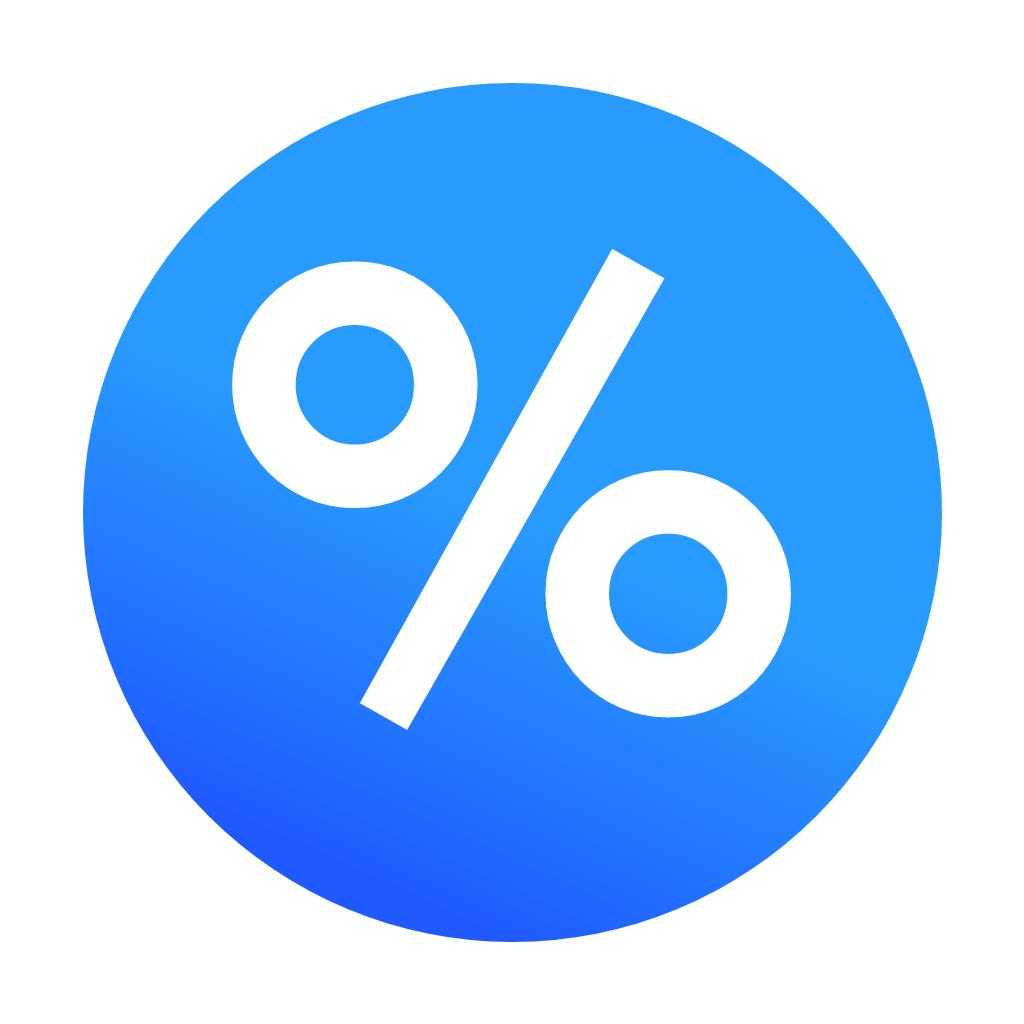Percentages Calculator 5 1140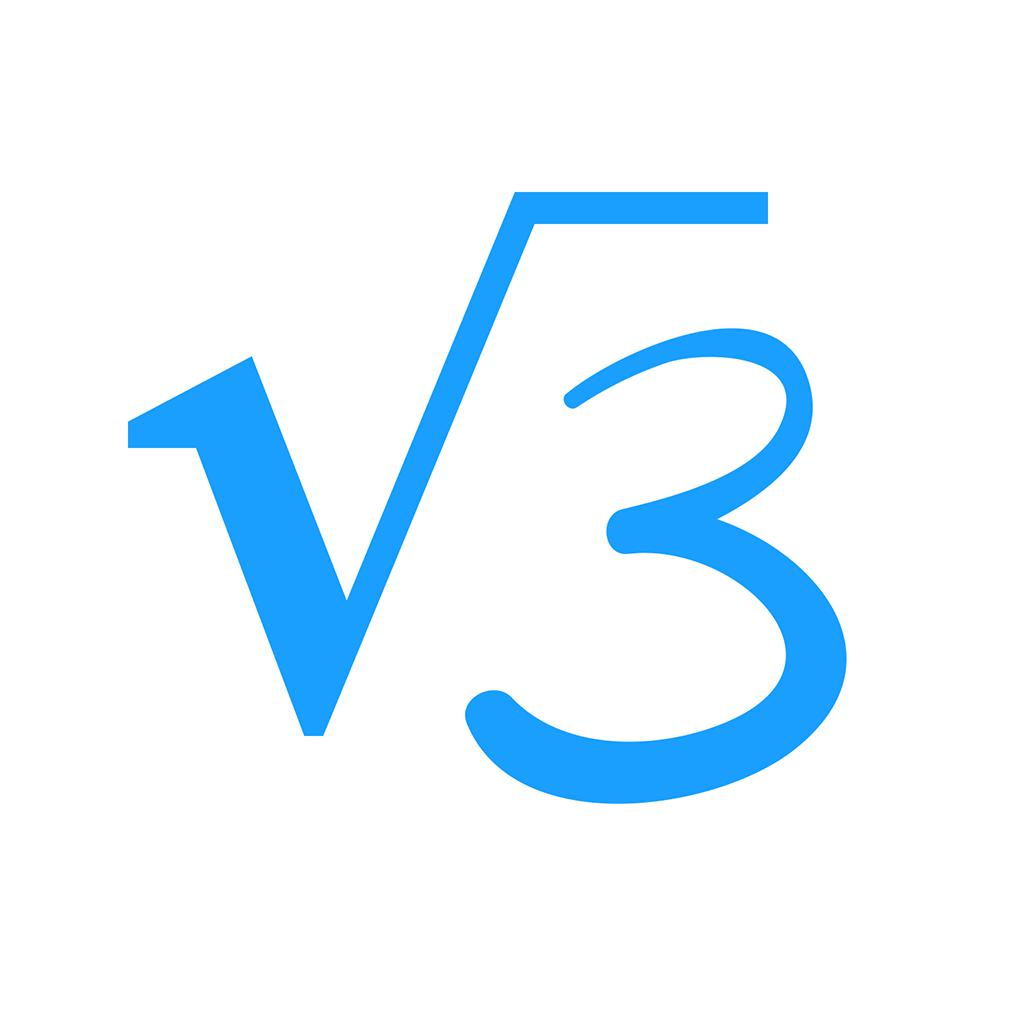MyScript Calculator-Handwriting calculator 4 654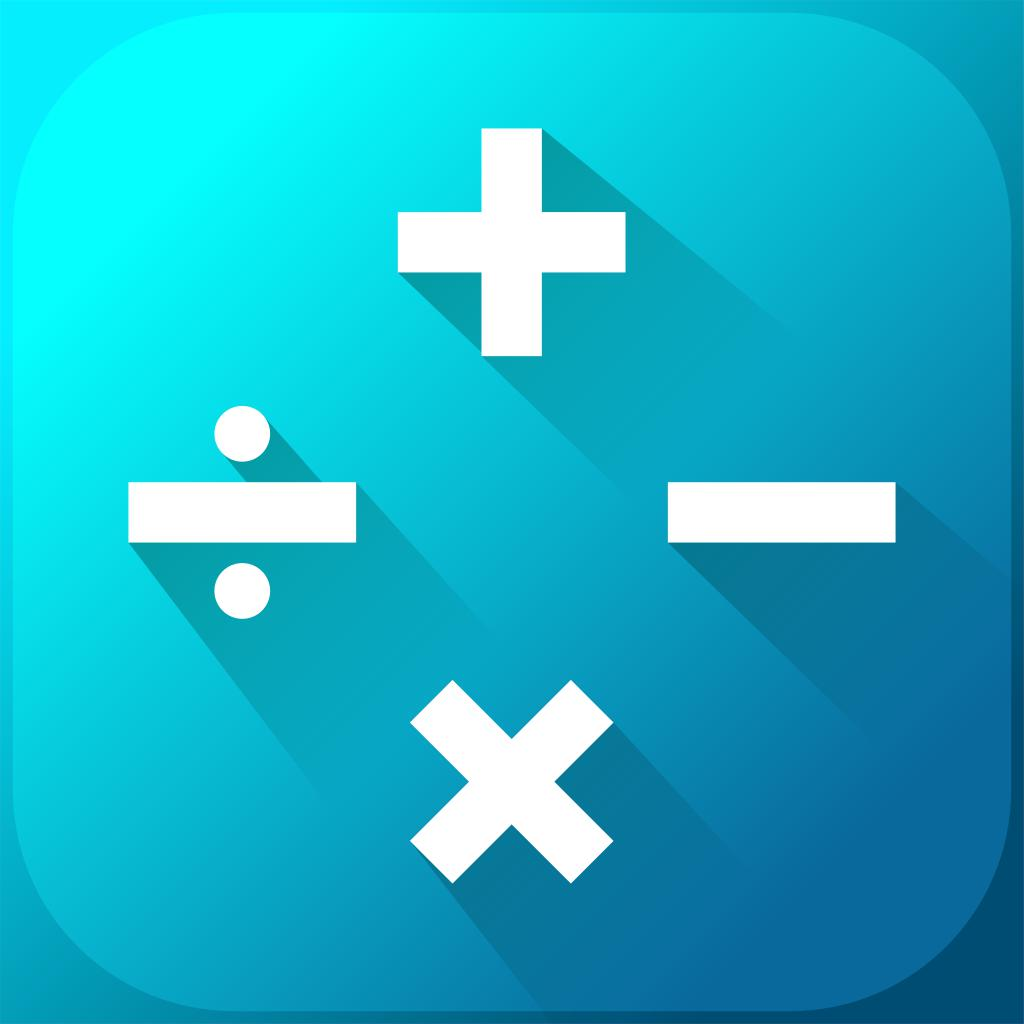Matix: Powerful math practice-Fun mental math training 5 104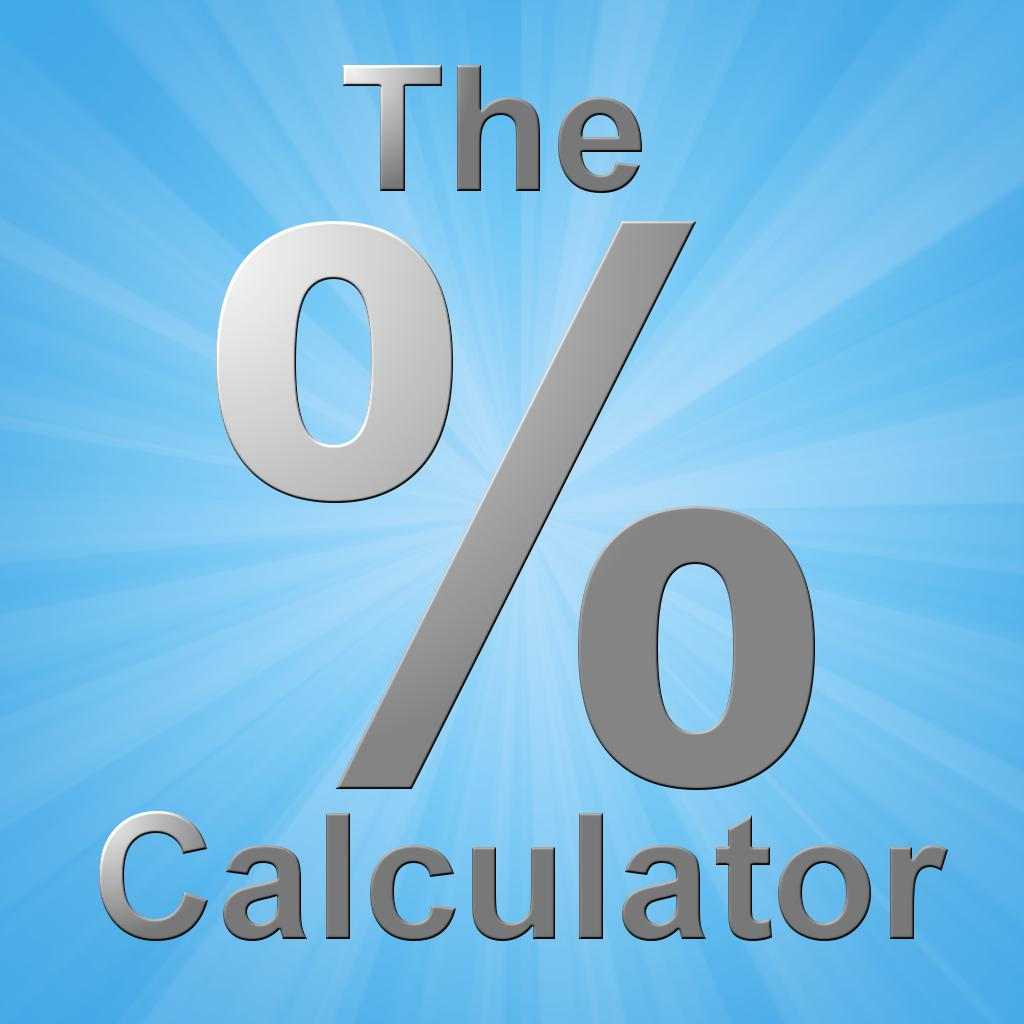The Percentage Calculator (Discount Calculator) 4 14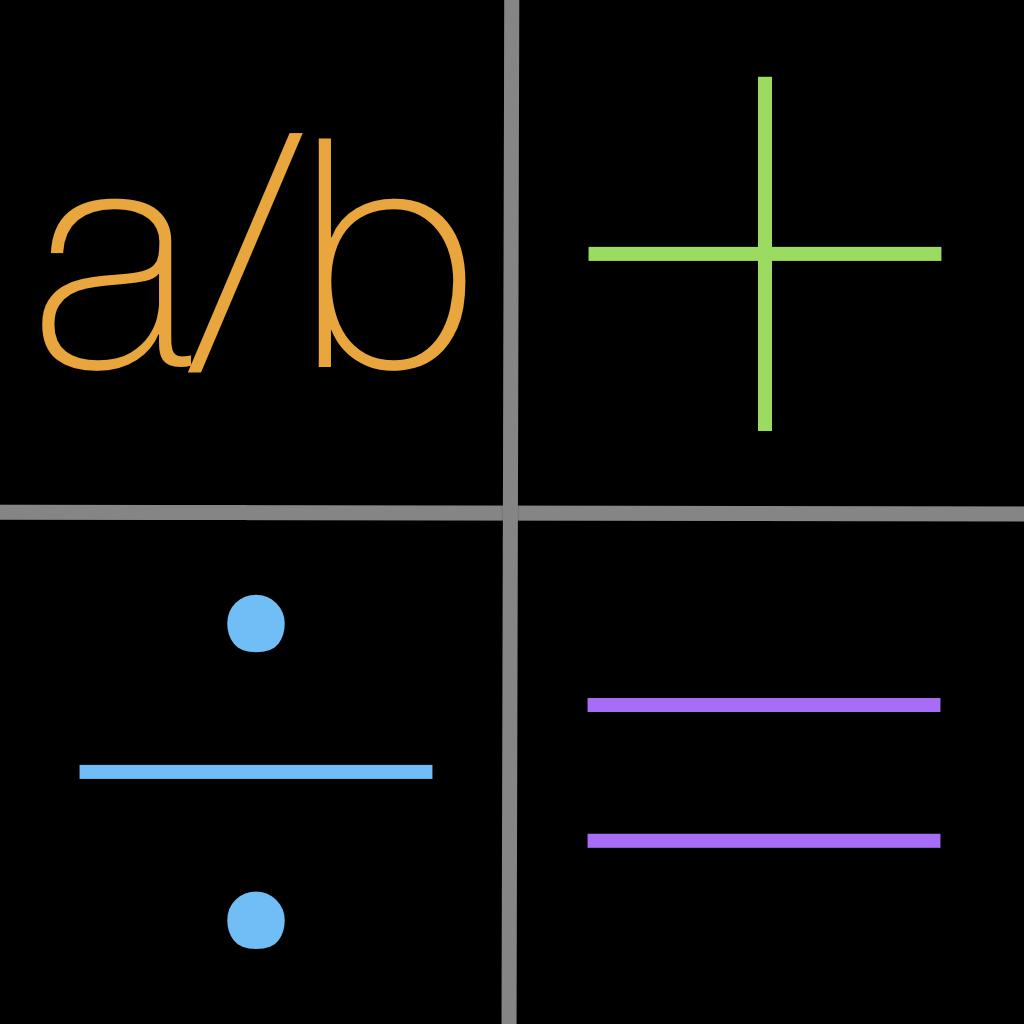jCalc - Multi Calculator Free — with a history of your calculations plus a fraction calculator for iPhone, iPad and iPod touch 4 115

"math pourcentages" has 77 apps. Top 5 are Percentages Calculator, MyScript Calculator, Matix: Powerful math practice, The Percentage Calculator (Discount Calculator), and jCalc - Multi Calculator Free — with a history of your calculations plus a fraction calculator for iPhone, iPad and iPod touch.

• %  Related Apps & App Analytics
 Apps Average Star Ratings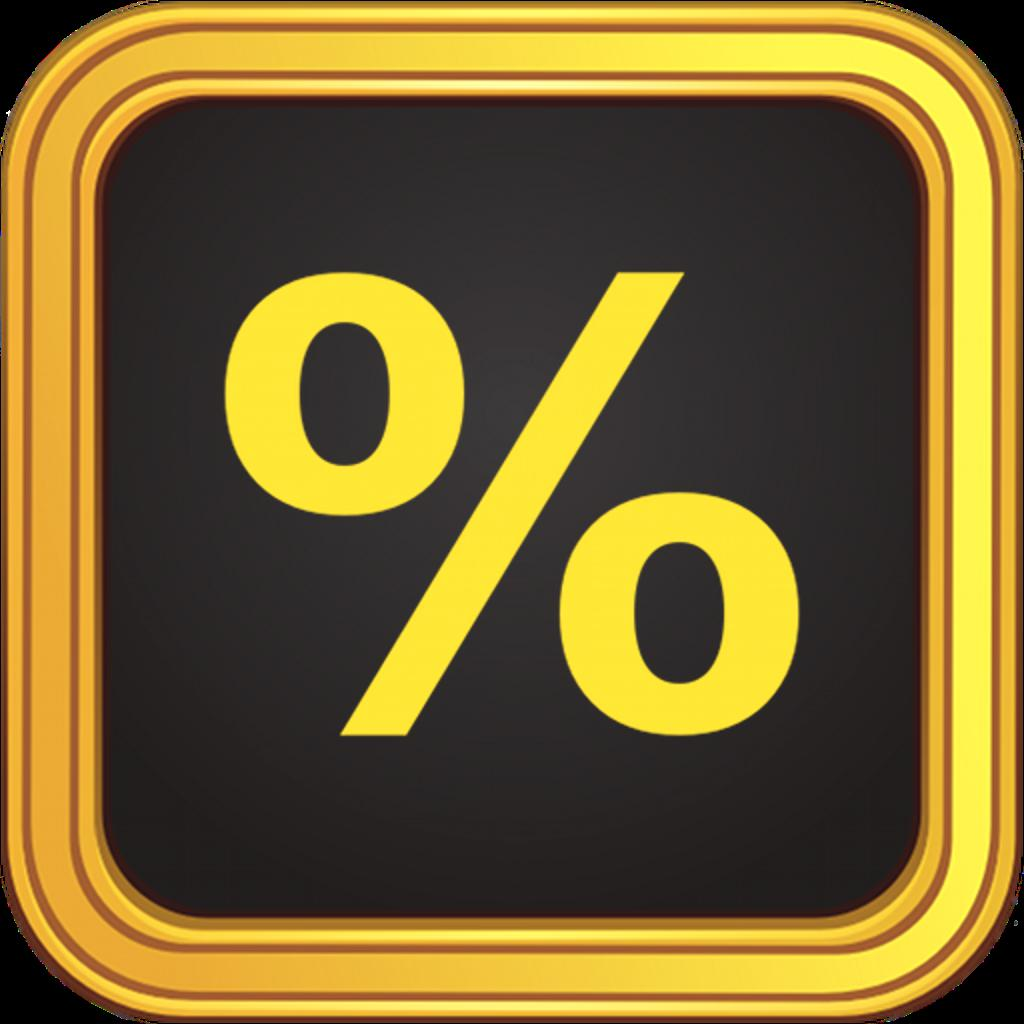Tip Calculator % Gold-Fast Tips & Split Bills App 5 17936Percentages Calculator 5 1140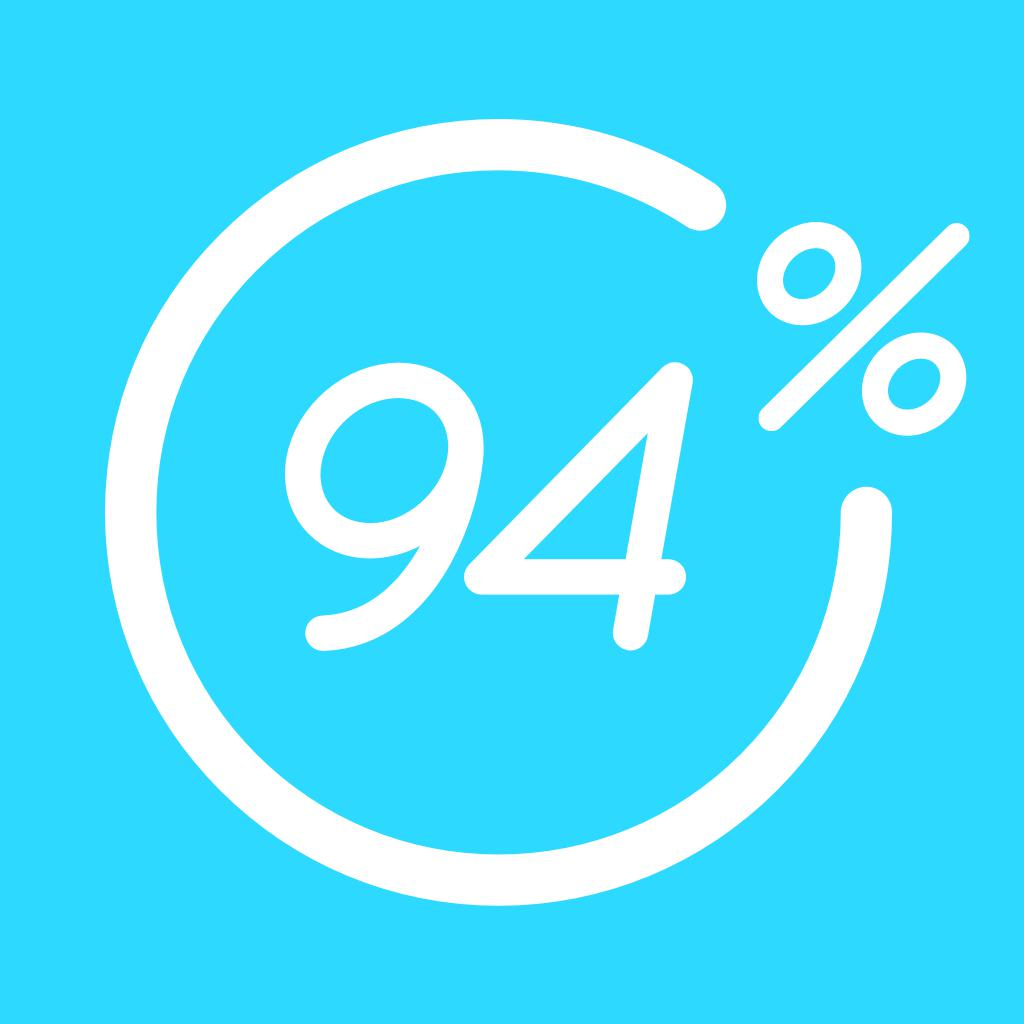94% - Quiz, Trivia & Logic-Test your knowledge! 3 2373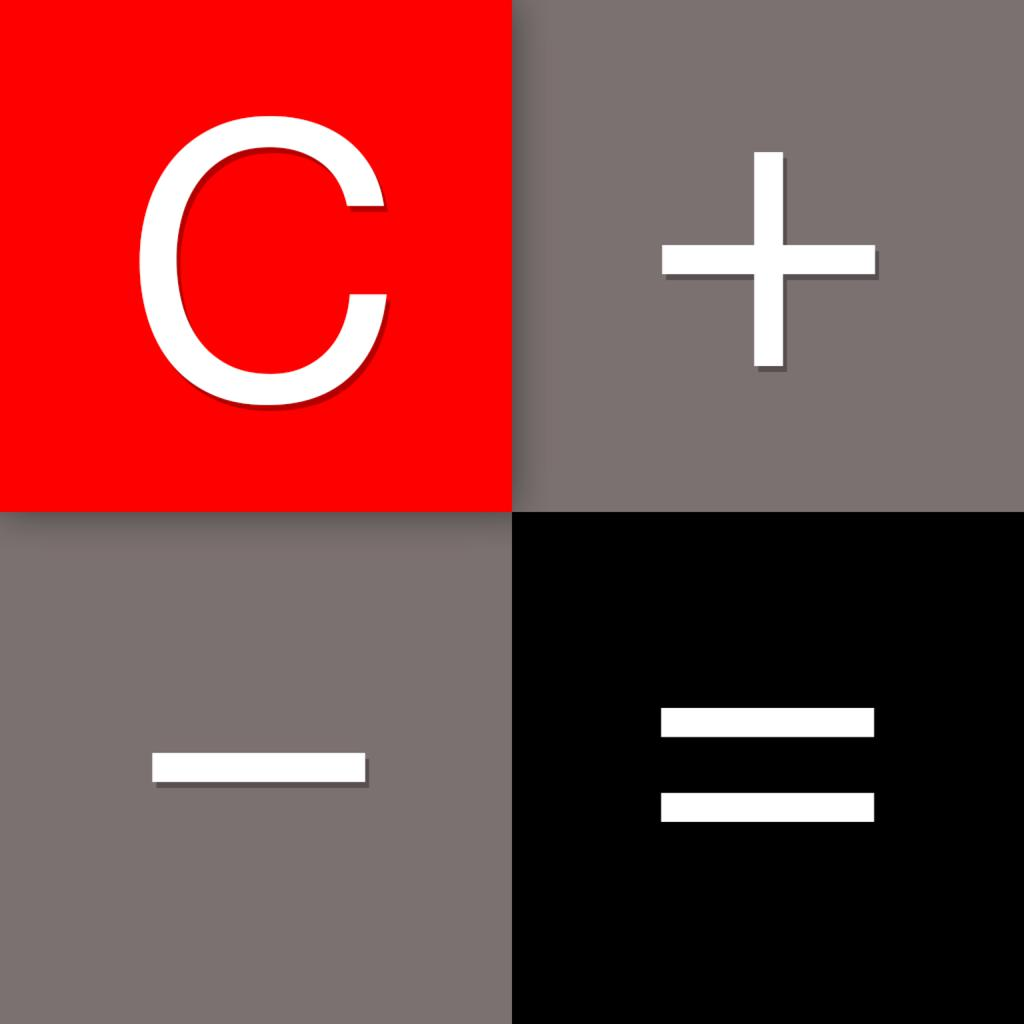Calculator‰-Default Calculator 5 299596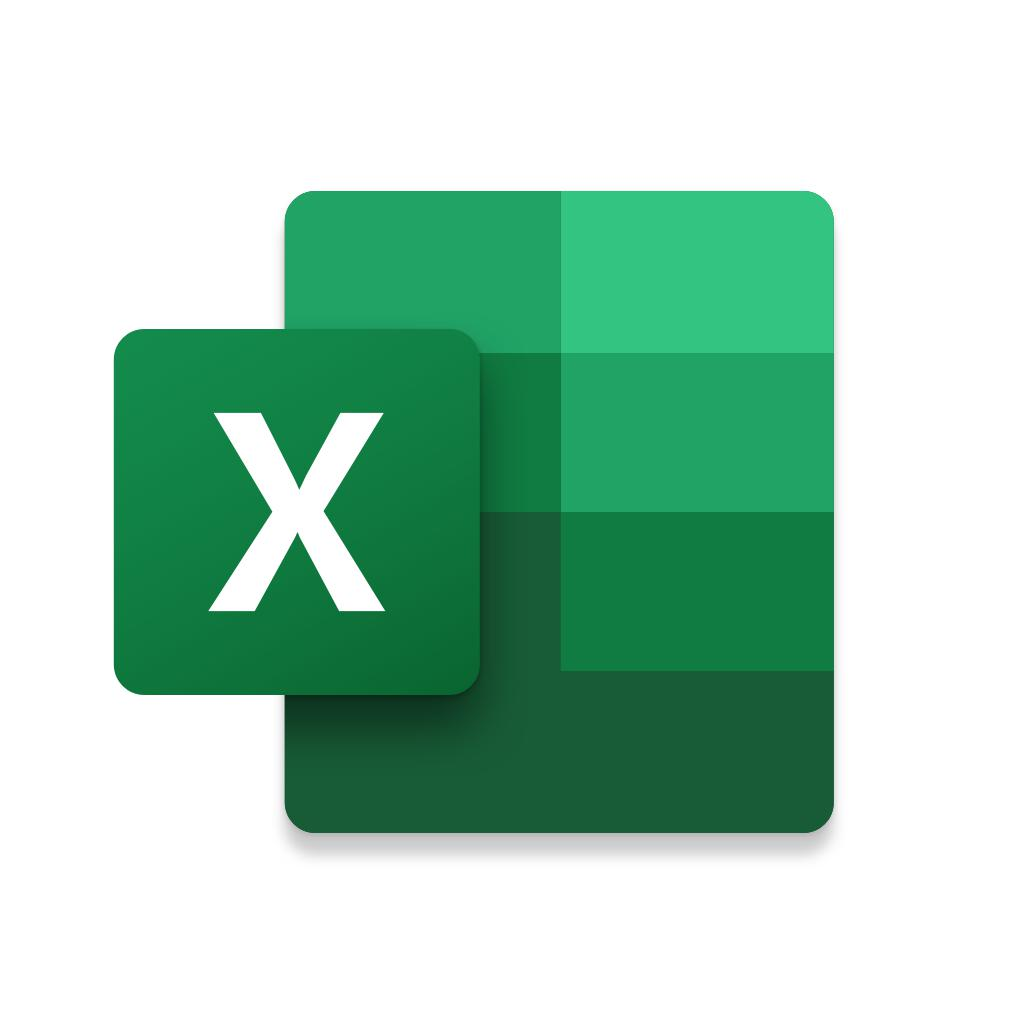Microsoft Excel-Charts, Data, Budget & Numbers 5 609538

"%" has 243 related apps in total. Top 5 are Tip Calculator % Gold, Percentages Calculator, 94% - Quiz, Trivia & Logic, Calculator‰, and Microsoft Excel.

• percentages calculator  Related Apps & App Analytics
 Apps Average Star Ratings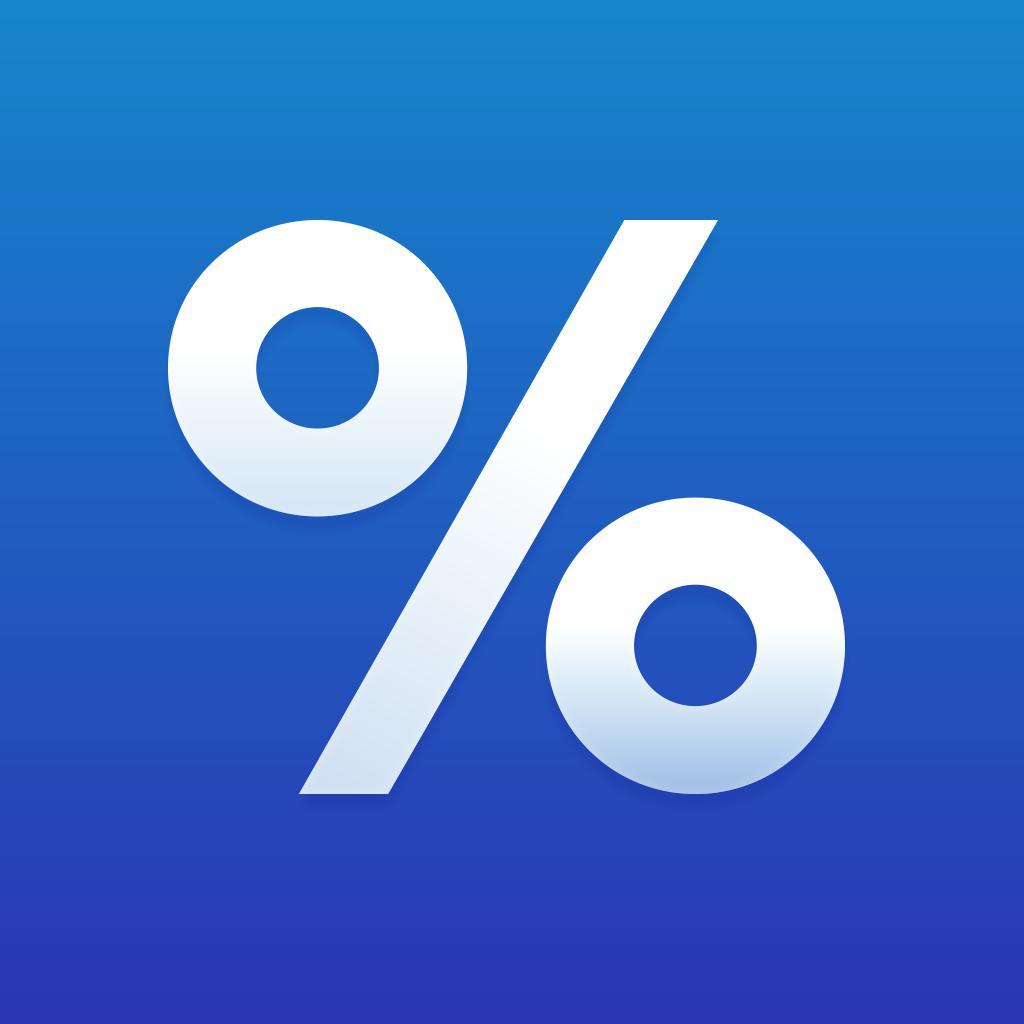Percentage Calculator ٞ-Calculate percents & fractions 4 3667Percentages Calculator 5 1140The Percentage Calculator (Discount Calculator) 4 14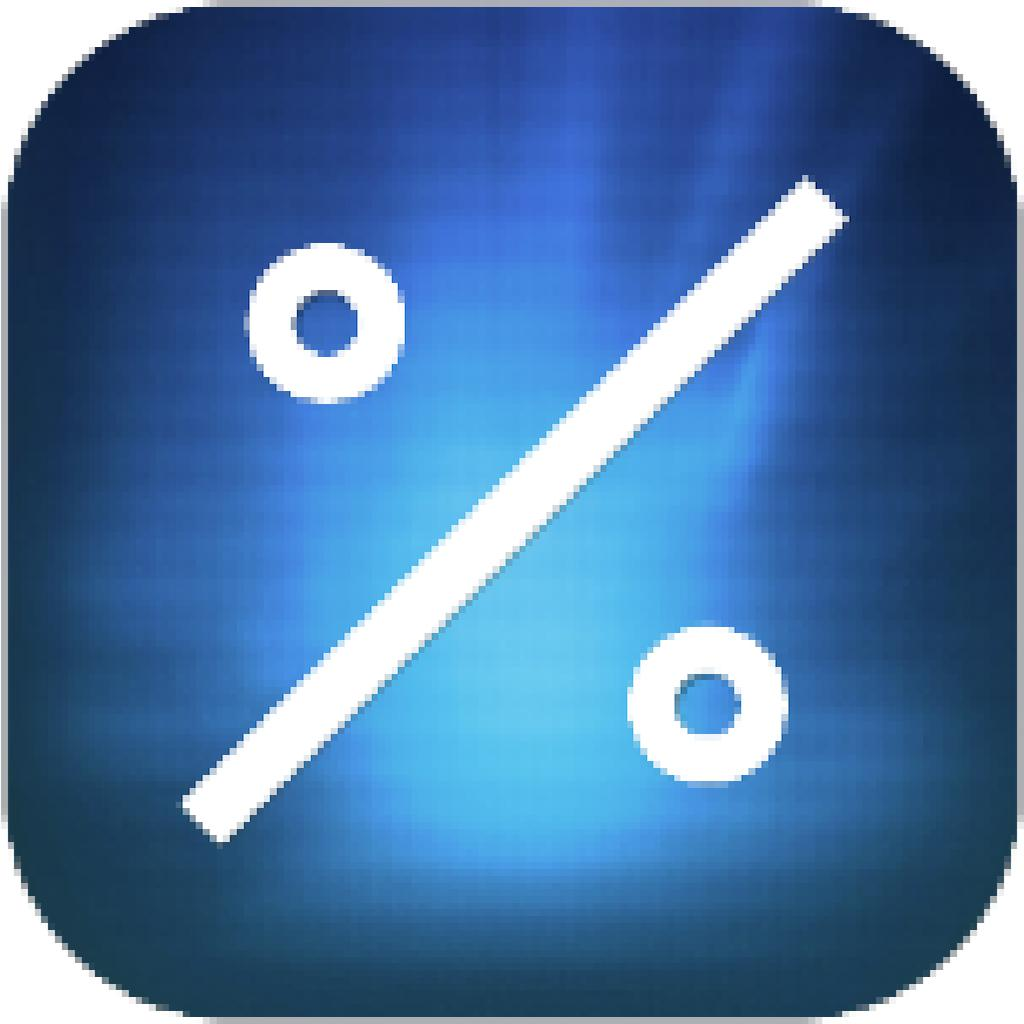Percentage Calculators 4 13Percentage Calculator - percent, discount, tip 4 40

"percentages calculator" has 192 related apps in app store. Top 5 are Percentage Calculator ٞ, Percentages Calculator, The Percentage Calculator (Discount Calculator), Percentage Calculators, and Percentage Calculator - percent, discount, tip.

• change calculator app  Related Apps & App Analytics
 Apps Average Star Ratings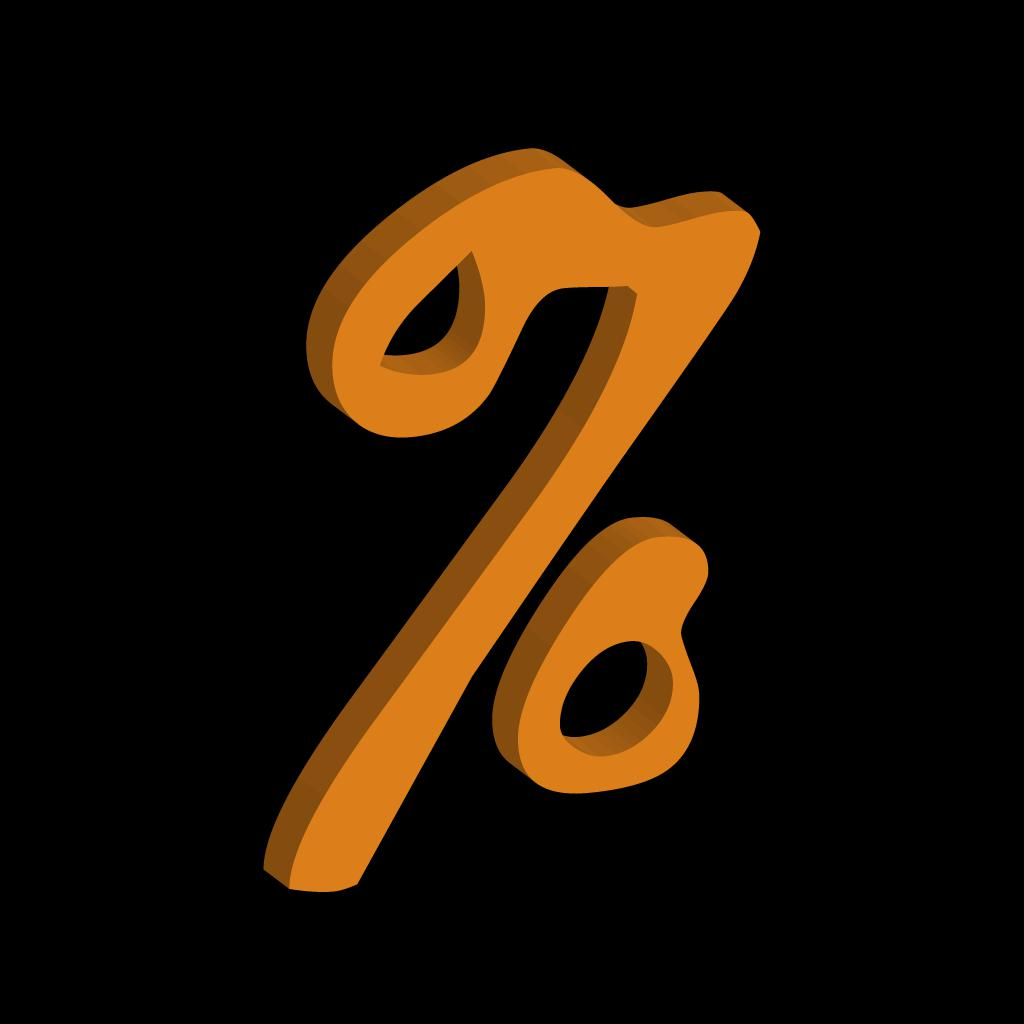PercentDiff - the Shaolo Percent and Percentage Calculator 5 116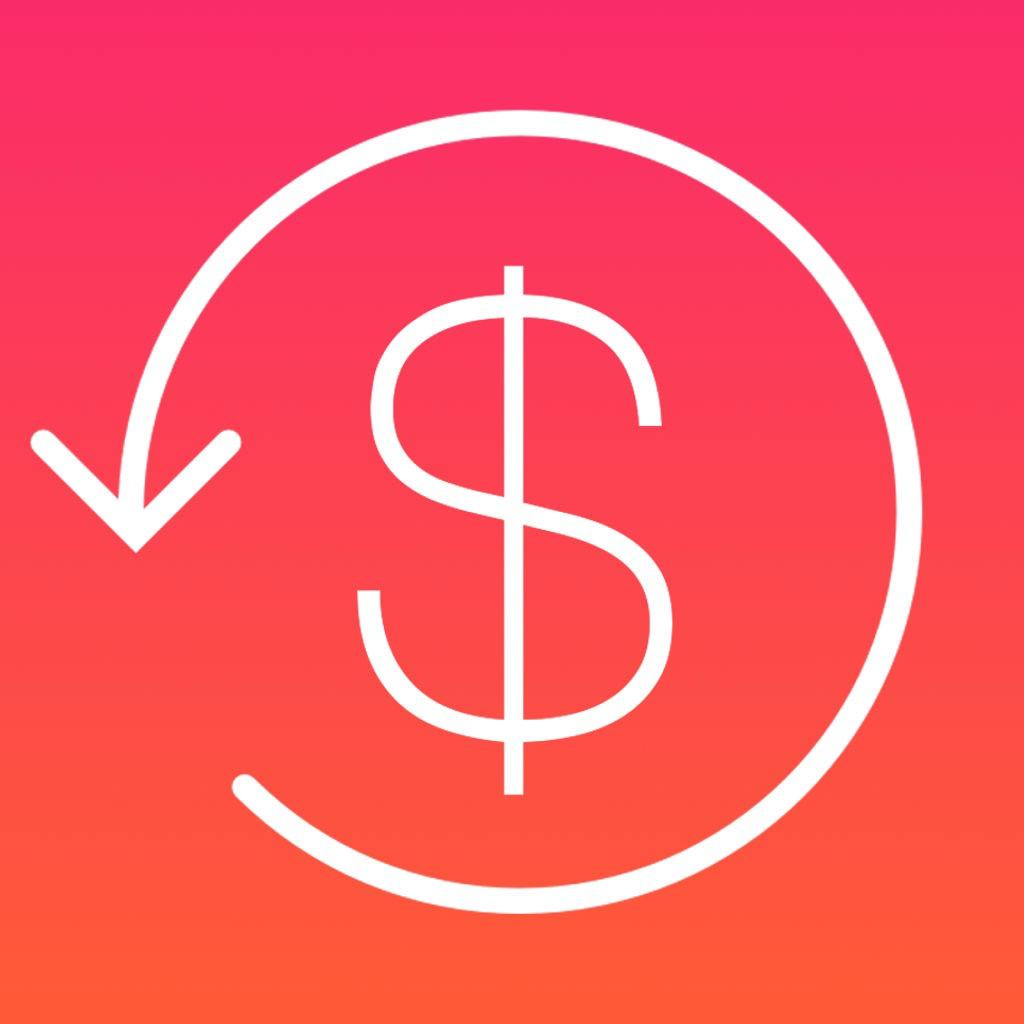Change Calculator-Calculate in seconds! 2 7Percentages Calculator 5 1140Elk Travel Currency Converter-Winner of ADA 2017 4 1069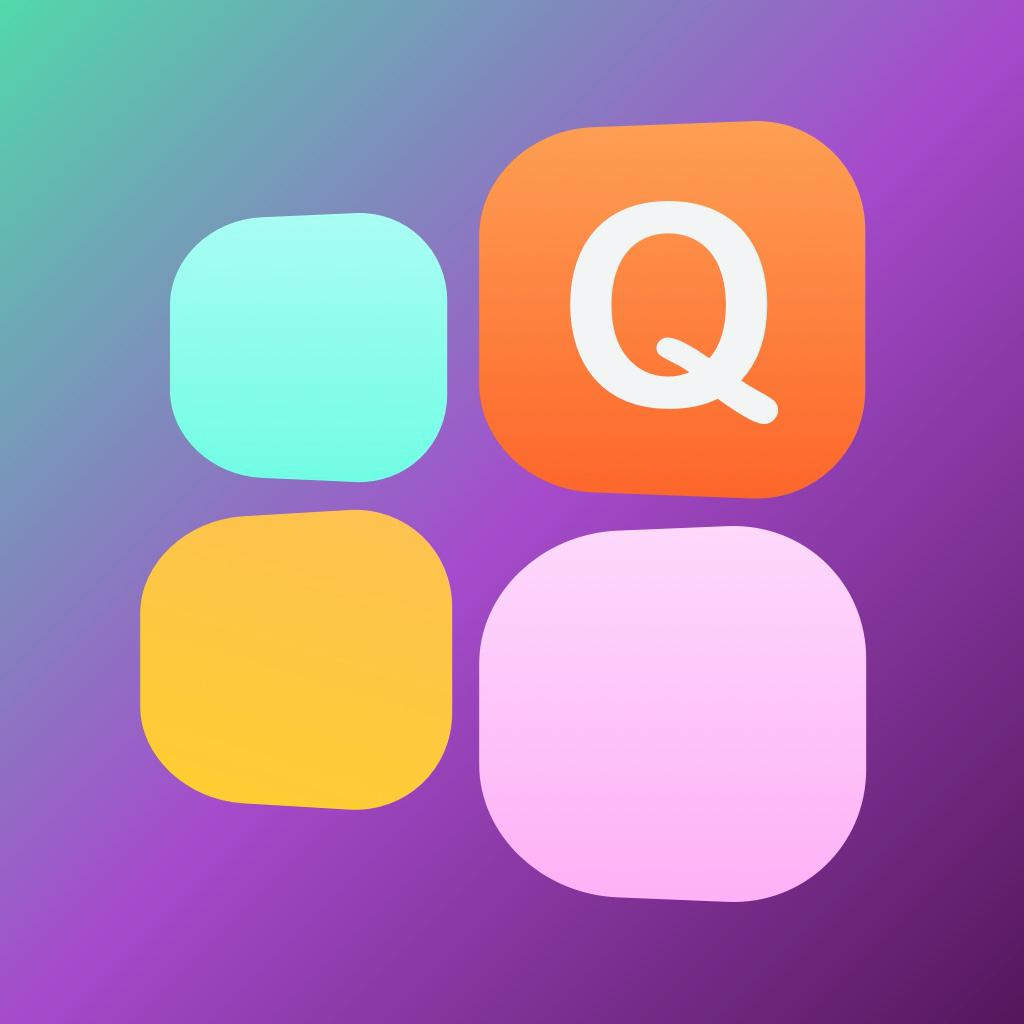Quote Widget-Edit home screen-Change color, font, theme 4 6

"change calculator app" has 96 related apps. Top 5 apps are PercentDiff - the Shaolo Percent and Percentage Calculator, Change Calculator, Percentages Calculator, Elk Travel Currency Converter, and Quote Widget-Edit home screen.

• percentage change calculator  Related Apps & App Analytics
 Apps Average Star RatingsDiff Calculator-Change percentage calculator 5 1PercentDiff - the Shaolo Percent and Percentage Calculator 5 116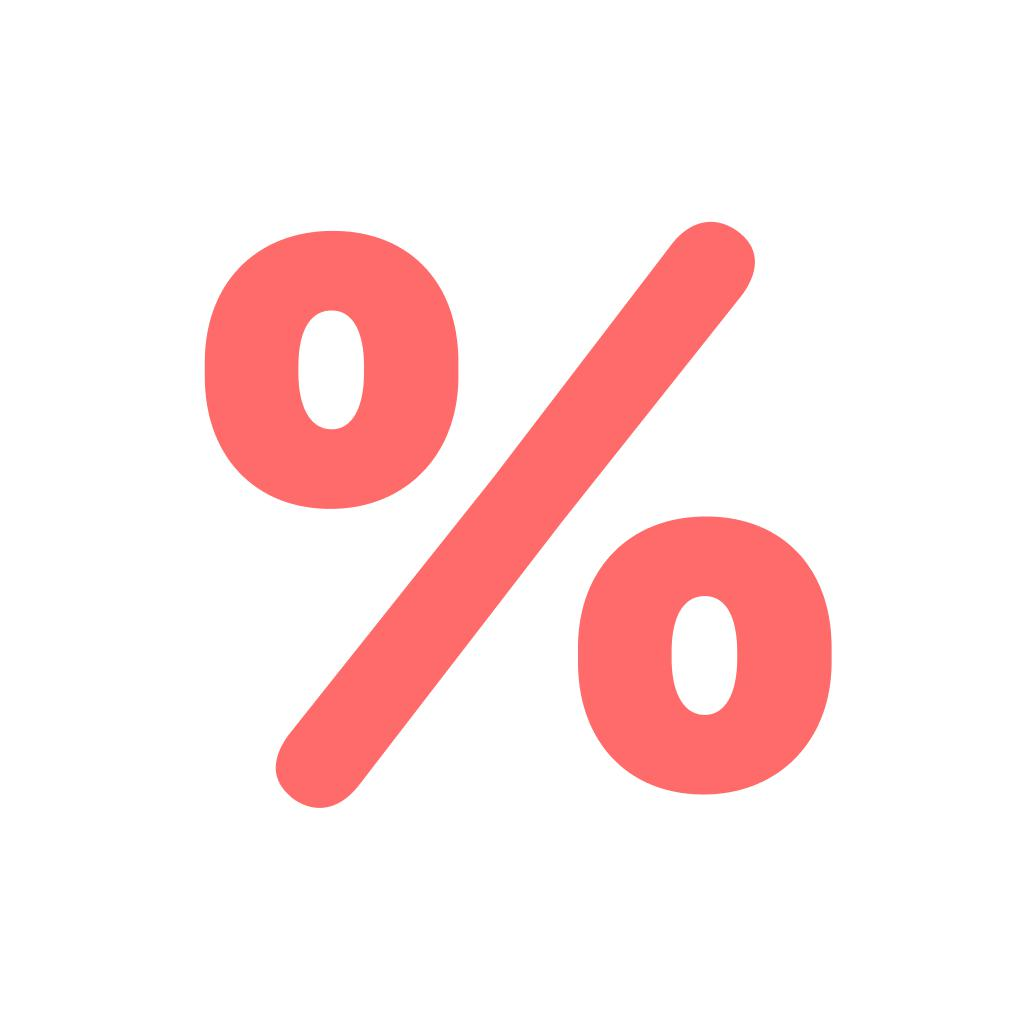% Calc - Percentage Calculator 5 1Percentages Calculator 5 1140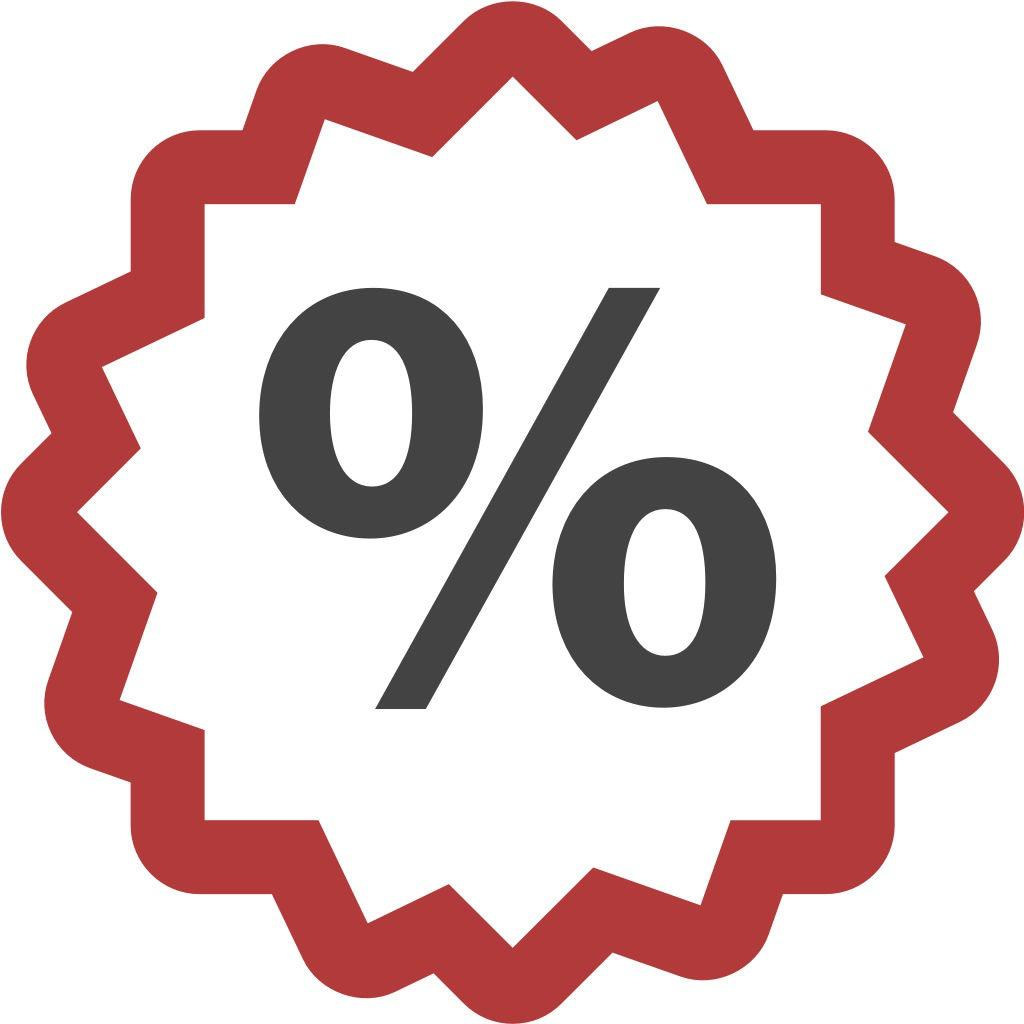Percentage Change Calculator 4 1

12 apps are ranking for "percentage change calculator" in app store. Top 5 apps are Diff Calculator, PercentDiff - the Shaolo Percent and Percentage Calculator, % Calc - Percentage Calculator, Percentages Calculator, and Percentage Change Calculator.

As you can see, Percentages Calculator, Percentages Calculator, and MyScript Calculator are ranking for math pourcentages, also, Percentages Calculator, Tip Calculator % Gold, Percentages Calculator and Calculator‰ are all competing the keyword %.

Before promoting your apps in app store, you'd better perform app analytics for better ASO tactics, and ASOTools, free ASO tools will help you with App store keywords optimization.